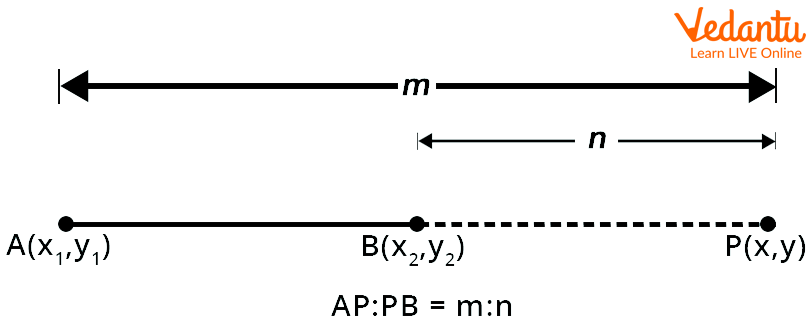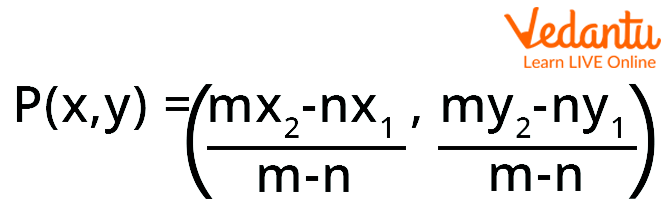Courses
Courses for Kids
Free study material
Free LIVE classes
More

# External Division FormulaLIVE
Join Vedantu’s FREE Mastercalss

## Introduction:

The line segment is divided into two pieces by a point, which may or may not be equal. We are able to determine the ratio by which the provided line segment is divided if we are aware of the point's coordinates.

## What is the Section Formula?

The coordinates of a point that separates a line segment externally or internally in a specific ratio can be found using the section formula. It's a helpful tool for figuring out the location of the point where a line segment is divided into a specific number of segments.

This section formula can be used to find the midpoint of a line segment as well as to derive the midpoint formula.

## External Division Formula:

When the line segment is divided externally by the point in the specified ratio, the external section formula is applied. The coordinates of the point on the line segment connecting the two points and falling beyond the two points, in the specified ratio, are found using this formula. Look at the following illustration to see how a point P(x, y) externally divides the line segment AB in a ratio such that ${\rm{AP:PB=m:n}}$.The coordinates of the point P are now stated as follows in terms of the ratio supplied and for the given coordinates of the points A$\left( {{x_1},{y_1}} \right)$ , B $\left( {{x_{2,}}{y_2}} \right)$:Section of a Line Dividing it in Ratio M : NSection Formula

where,

The coordinates for point P are (x,y)

Point A's coordinates are $\left( {{x_1},{y_1}} \right)$.

The coordinates of point B is $\left( {{x_{2,}}{y_2}} \right)$.

The ratio values at which P divides the line externally are m and n.

Difference between Internal and External Division Formula:

This formula can be used when a point internally divides a line segment in the ratio m: n at point C and that point lies between the coordinates of the line segment. Internal Division is another name for it.Internal Section FormulaExternal Division formula

We can use this formula when the point that splits the line segment is divided externally in the ratio m: n sits outside the line segment, which means that when we extend the line, the point corresponds with the line. It also goes by the name External Division.

## Conclusion :

We can use this formula when the point that splits the line segment is divided externally in the ratio m: n sits outside the line segment, which means that when we extend the line, the point corresponds with the line. It also goes by the name External Division.

## Solved Examples:

1. Find the ratio in which a point P lying on the y-axis divides the line joining points (6, -6) and (-2, -4).

Let , ratio be $m:n$ .

Since the point P lies on the y-axis, the y-axis will be 0 and let us assume the x-axis to be 'a'.

So, according to the formula let us take$\left( {6, - 6} \right) = \left({{x_1},{y_1}} \right)$

$\left[ { - 2, - 4} \right] = \left[ {{x_2},{y_2}} \right]$

and $\left[ {0,a} \right] = \left[ {x,y} \right]$

$x = \dfrac{{m{x_2} + n{x_1}}}{{m + n}}$

$\begin{array}{l}0 = - 2m + 6n\\2m = 6n\\m/n = 3/1\\m:n = 3:1\end{array}$

2. What does the formula for internal and external sections mean?

The section formula aids in pinpointing a point's coordinates, making it easier to divide the line connecting two locations into ratios. Either internally or externally, something occurs. Following is the formula:

$x = \dfrac{{m{x_2} + n{x_1}}}{{m + n}}$

3. Using the section formula, determine the coordinates of the point C that externally divides the points A (-3, -1), and B (-1, 0) in a ratio of 2:3.

Given,

$\left[ {{x_1},{y_1}} \right] = \left[ { - 3, - 1} \right]$

$\left( {{x_2},{y_2}} \right) = \left( { - 1,0} \right)$

$m:n = 2:3$

the location of the point C's coordinates (x, y)

Using the external division formula for sections,

$\begin{array}{l}C\left[ {x,y} \right] = \left[ {\dfrac{{\left[ {2\left[ { - 1} \right] - 3\left[ { - 3} \right]} \right]}}{{2 - 3}},\dfrac{{\left[ {2\left[ 0 \right] - 3\left[ { - 1} \right]} \right]}}{{2 - 3}}} \right]\\{\text{After solving we will get:}}\\C\left( {x,y} \right) = \left( { - 7, - 3} \right)\end{array}$

Thus, the coordinate of point C is (-7, -3)

Last updated date: 29th Sep 2023
Total views: 68.4k
Views today: 0.68k

## FAQs on External Division Formula

1. Why is the ratio in the exterior division negative?

When the divisor and dividend have opposite signs, a ratio is considered negative.

2. The definition of external division

Suppose P divides AB externally in the ratio m:n if P=(x,y) lies on the extension of line segment AB (not between points A and B) and fulfills ${\rm{AP:PB = m:n}}$

3. Why is a section formula necessary?

The coordinate of a point that splits a line segment connecting two points into two halves with a length ratio of m:n is found using the section formula.

4. What's the formula for the section?

The section formula assists in locating a point's coordinates, making it easier to divide the line connecting two points into equal parts.

5. What is a line segment's division?

Any natural number, 'n,' can be used to divide a line segment into an equal number of parts.